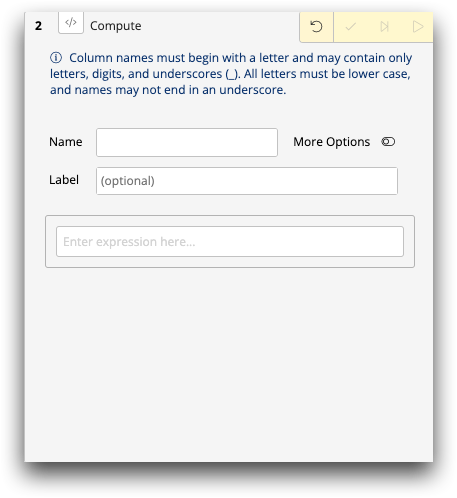# Computed column

A computed column is a column that you add to a table, whose values are typically based on one or more existing columns.

A computed column is determined by a given value expression. The value expression may refer to one or more columns and may include standard arithmetic, relational, and logical operators.

To create a column in the Trillion-Row Spreadsheet, use the Expression Editor in the Computed column panel. For more information about the Expression Editor, see Expression Editor.Figure: Computed column panel

For example, suppose a table has the columns Sales and Cost. You can create a new column Margin, which is Sales minus Cost.

In the Expression Editor, the value expression to create this computed column would be:

``sales-cost``
Note: Column names (e.g., sales), not column headings (e.g., Sales) are used in value expressions.

You can also create a computed column using the 1010data Insights Platform Macro Language XML code. Macro Language code can be entered in the Macro Language view of the Computed column panel and in the Macro Language Workshop.

In the Macro Language, the code to create this same computed column would be:

``<willbe name="margin" value="sales - cost" label="Margin"/>``

In addition to simple arithmetic operators, there are more advanced mathematical functions, functions that perform mathematical operations on dates (e.g., find the number of days between the dates in two columns), functions to manipulate strings, and categorization functions (e.g., is the value in the column between 0 and 10, between 10 and 100, or greater than 100?).

For example, to find the sum of sales for each store in the Sales Item Detail table, you can create a computed column that uses the G_Function `g_sum(G;S;X)`. In Macro Language, the code to create this computed column would be:

``<willbe name="sum_of_sales" value="g_sum(store;;sales)" label="Sum of Sales"/>``

In this example, `G` is the column we're grouping on (in our example, store); `S` is a selection column (by omitting a value for this, we're telling the G_Function to select all rows when computing the sum); and `X` is the column we are acting on (in our example, we want the sum of sales).

An important feature of computed columns is that missing ("N/A") values are handled automatically. For example, if Sales is N/A on one or more rows, then Margin (Sales minus Cost) will also be N/A on those rows. See the description of each operator and function for details about the way it handles N/A values.